## 重整化微扰论

1. 引入正规化方法，得到一个依赖于裸参数 $m_0,e_0$ 以及截断 $\Lambda$ 的值。

2. 计算物理质量 $m$ 与物理电荷量 $e$。若想要计算散射矩阵，还需要计算场强重整化常数 $Z$

3. 将这些表达式结合起来，用 $e,m$ 消去 $e_0,m_0$，这个步骤被称为重整化。

### 标量场的重整化理论

#### $\phi^4$ 理论的重整化

$\mathcal{L} = \frac{1}{2}(\partial_{\mu}\phi)^2 - \frac{1}{2}m_0^2 \phi^2 -\frac{\lambda_0}{4!}\phi^4 \tag{1}$

$D = 4- N \tag{2}$

• $N = 0$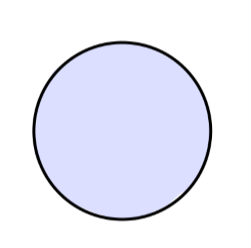对应真空，不可观测

• $N = 2$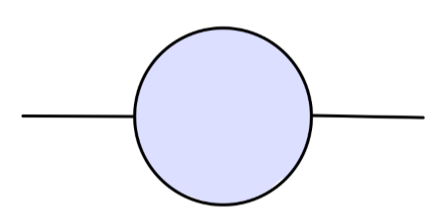$\sim \Lambda^2 + p^2\log \Lambda + (finite\ terms)$

• $N = 4$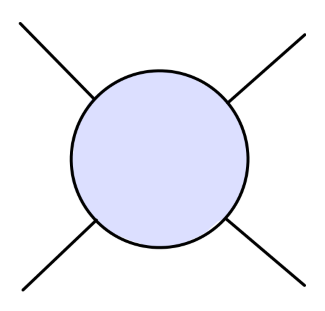$\sim \log \Lambda + (finite\ terms)$

$\int d^4x \langle \Omega| T\phi(x)\phi(0)|\Omega\rangle e^{ip\cdot x} \sim \frac{iZ}{p^2-m^2}$

$\phi = Z^{1/2}\phi_r \tag{3}$

$\mathcal{L} = \frac{1}{2}Z(\partial_{\mu}\phi_r)^2 - \frac{1}{2}m_0^2 Z\phi_r^2 -\frac{\lambda_0}{4!}Z^2\phi_r^4 \tag{4}$

\begin{aligned} \delta_Z &= Z-1\\ \delta_m &= m_0^2Z-m^2\\ \delta_\lambda &= \lambda_0 Z^2-\lambda\\ \end{aligned}\tag{5}

\begin{aligned} \mathcal{L} =& \frac{1}{2}(\partial_{\mu}\phi_r)^2 - \frac{1}{2}m^2\phi_r^2 - \frac{\lambda}{4!}\phi_r^4 \\ & + \frac{1}{2}\delta_Z (\partial_{\mu}\phi_r)^2-\frac{1}{2}\delta_m\phi_r^2 - \frac{\delta_\lambda}{4!}\phi_r^4\tag{6} \end{aligned}$\frac{i}{p^2 - m^2+ i\epsilon}$$-i\lambda$$i(p^2\delta_Z -\delta_m)$$-i\delta_{\lambda}$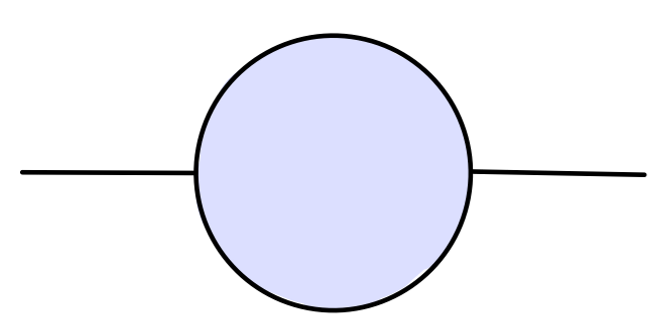$\frac{i}{p^2-m^2} + (terms\ regular\ at\ p^2 = m^2)$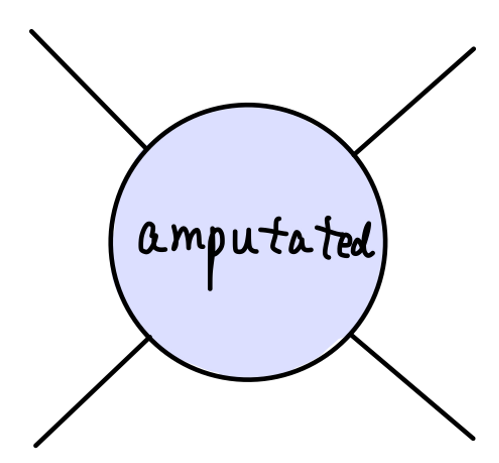$-i\lambda \quad at\ s = 4m^2,\ t=u=0$

#### $\phi^4$ 重整化理论的一阶圈图计算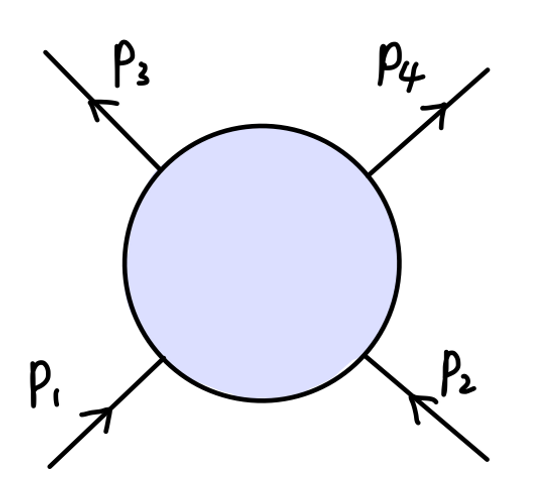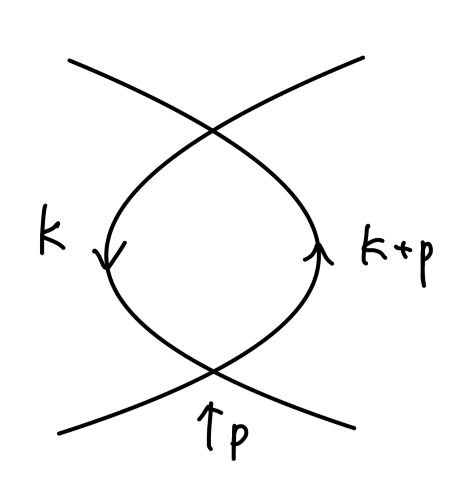\begin{aligned} \frac{(-i\lambda)^2}{2}\int \frac{d^4k}{(2\pi)^4} \frac{i}{k^2-m^2}\frac{i}{(k+p)^2-m^2} \equiv (-i\lambda)^2\cdot iV(p^2) \end{aligned}

$(-i\lambda)^2iV(t)\quad and\quad (-i\lambda)^2iV(u)$

counterterm 的值为：

$-i\delta_{\lambda}$

$i\mathcal{M} = -i\lambda + (-i\lambda)^2[iV(s)+iV(t)+iV(u)] - i\delta_{\lambda} \tag{7}$

$-i\lambda + (-i\lambda)^2[iV(4m^2)+iV(0)+iV(0)] - i\delta_{\lambda}=-i\lambda$

$\delta_{\lambda} = -\lambda^2[V(4m^2)+2V(0)] \tag{8}$

\begin{aligned} V(p^2) &= \frac{-i}{2}\int \frac{d^d k}{(2\pi)^d} \frac{i}{k^2-m^2}\frac{i}{(k+p)^2-m^2}\\ &= \frac{i}{2}\int_{0}^{1}dx \int \frac{d^d k}{(2\pi)^d} \frac{1}{[k^2 + 2xk\cdot p + xp^2-m^2]^2}\\ &\underset{l=k+xp}{=} \frac{i}{2}\int_{0}^{1}dx \int \frac{d^d l}{(2\pi)^d} \frac{1}{[l^2 + x(1-x)p^2-m^2]^2}\\ &\underset{l_E^0 = -il^0}{=} -\frac{1}{2}\int_{0}^{1}dx \int \frac{d^d l_E}{(2\pi)^d} \frac{1}{[l_E^2 - x(1-x)p^2+m^2]^2}\\ &= -\frac{1}{2}\int_{0}^{1}dx \frac{1}{(4\pi)^{d/2}}\Gamma(2-\frac{d}{2})\frac{1}{(m^2-x(1-x)p^2)^{2-d/2}}\\ & \underset{d\rightarrow 4}{\longrightarrow} -\frac{1}{32\pi^2}\int_{0}^{1}dx (\frac{2}{\epsilon} - \gamma + \log (4\pi) - \log [m^2-x(1-x)p^2])\\ \end{aligned}

\begin{aligned} \delta_{\lambda} &= -\lambda^2[V(4m^2)+2V(0)]\\ &= \frac{\lambda^2}{2}\int_{0}^{1}dx \frac{\Gamma(2-\frac{d}{2})}{(4\pi)^{d/2}}[\frac{1}{(m^2-x(1-x)4m^2)^{2-d/2}} + \frac{2}{(m^2)^{2-d/2}}]\\ & \underset{d\rightarrow 4}{\longrightarrow} \frac{\lambda^2}{32\pi^2}\int_{0}^{1}dx (\frac{6}{\epsilon} -3\gamma +3\log 4\pi - \log[m^2-x(1-x)4m^2] - 2\log[m^2])\\ \end{aligned}

$d\rightarrow 4$ 时，上述结果是发散的，这没有关系，$\delta_{\lambda}$ 并不是一个可观测量。我们将 $\delta_{\lambda}$ 代回到 $(7)$ 式，将得到一个有限值：

\begin{aligned} i\mathcal{M} &= -i\lambda + (-i\lambda)^2[iV(s)+iV(t)+iV(u)] - i\delta_{\lambda}\\ &= -i\lambda - \frac{i\lambda^2}{32\pi^2}\int_{0}^{1}dx[\log(\frac{m^2-x(1-x)s}{m^2-x(1-x)4m^2}) + \log(\frac{m^2-x(1-x)t}{m^2}) + \log(\frac{m^2-x(1-x)u}{m^2})]\\ \end{aligned}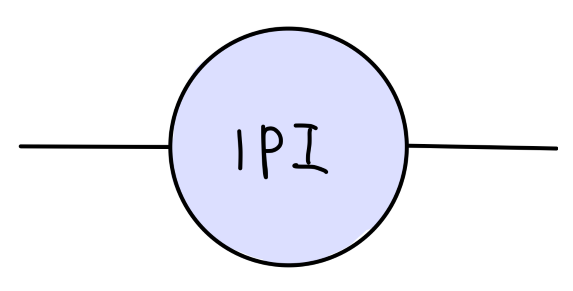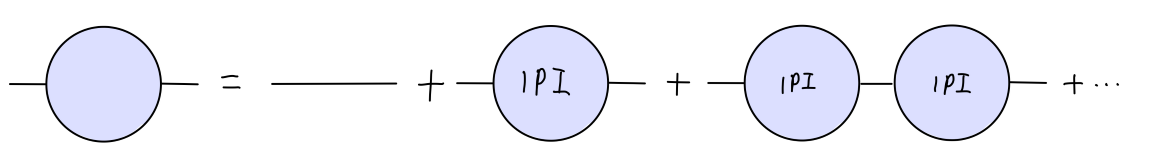$\frac{i}{p^2 - m^2 - M^2(p^2)}$

$M^2(p^2)|_{p^2=m^2} = 0 \quad and\quad \frac{d}{dp^2}M^2(p^2)|_{p^2=m^2} = 0\tag{9}$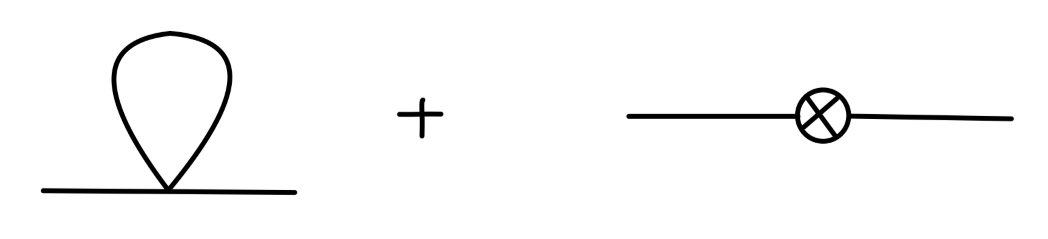\begin{aligned} -iM^2(p^2)& = -i\lambda\cdot\frac{1}{2}\cdot\int\frac{d^dk}{(2\pi)^d}\frac{i}{k^2-m^2} + i(p^2\delta_Z-\delta_m)\\ &= -\frac{i\lambda}{2} \frac{1}{(4\pi)^{d/2}}\frac{\Gamma(1-\frac{d}{2})}{(m^2)^{1-d/2}} + i(p^2\delta_Z-\delta_m)\\ \end{aligned}

$\delta_Z = 0\quad \delta_m = -\frac{\lambda}{2(4\pi)^{d/2}}\frac{\Gamma(1-\frac{d}{2})}{(m^2)^{1-d/2}}\tag{10}$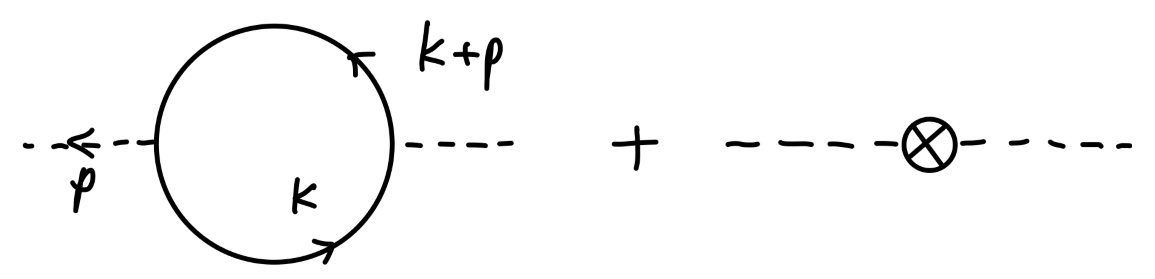\begin{aligned} -iM^2(p^2) &= -(-ig)^2\int \frac{d^dk}{(2\pi)^d}\mathrm{tr}[\frac{i(\cancel{k}+\cancel{p}+m_f)}{(k+p)^2-m_f^2}\frac{i(\cancel{k}+m_f)}{k^2-m_f^2}] + i(p^2\delta_Z - \delta_m)\\ &= -4g^2 \int \frac{d^dk}{(2\pi)^d} \frac{(k+p)\cdot k + m_f^2}{((k+p)^2-m_f^2)(k^2-m_f^2)} + i(p^2\delta_Z -\delta_m)\\ \end{aligned}

\begin{aligned} &-4g^2 \int \frac{d^dk}{(2\pi)^d} \frac{(k+p)\cdot k + m_f^2}{((k+p)^2-m_f^2)(k^2-m_f^2)}\\ =&-4g^2\int_{0}^{1}dx\int \frac{d^dk}{(2\pi)^d} \frac{(k+p)\cdot k + m_f^2}{(k^2 + 2xk\cdot p + xp^2 - m_f^2)^2}\\ \underset{l=k+xp}=&-4g^2\int_{0}^{1}dx\int \frac{d^dl}{(2\pi)^d} \frac{(l + (1-x)p)(l - xp) + m_f^2}{(l^2 + x(1-x)p^2 - m_f^2)^2}\\ =&-4g^2\int_{0}^{1}dx\int \frac{d^dl}{(2\pi)^d} \frac{l^2 -x(1-x)p^2 + m_f^2}{(l^2 + x(1-x)p^2 - m_f^2)^2}\\ =&-4g^2\int_{0}^{1}dx\frac{-i}{(4\pi)^{d/2}}(\frac{\frac{d}{2}\Gamma(1-\frac{d}{2})}{\Delta^{1-d/2}}-\frac{\Delta\Gamma(2-\frac{d}{2})}{\Delta^{2-d/2}})\\ =&\frac{4ig^2(d-1)}{(4\pi)^{d/2}}\int_{0}^{1}dx\frac{\Gamma(1-\frac{d}{2})}{\Delta^{1-d/2}}\\ \end{aligned}

$\delta_m = \frac{4g^2(d-1)}{(4\pi)^{d/2}}\int_{0}^{1}dx \frac{\Gamma(1-\frac{d}{2})}{[m_f^2 - x(1-x)m^2]^{1-d/2}} + m^2\delta_Z$

\begin{aligned} -i\frac{dM^2(p^2)}{dp^2}|_{p^2=m^2} &= \frac{4ig^2(d-1)}{(4\pi)^{d/2}}\int_{0}^{1}dx x(1-x)\frac{\Gamma(2-\frac{d}{2})}{\Delta^{2-d/2}} + i\delta_Z = 0 \end{aligned}

\begin{aligned} \frac{dM^2(p^2)}{dp^2}|_{p^2=m^2} &= -\frac{4g^2(d-1)}{(4\pi)^{d/2}}\int_{0}^{1}dx \frac{x(1-x)\Gamma(2-\frac{d}{2})}{(m_f^2 - x(1-x)p^2)^{2-d/2}}\\ &\underset{d\rightarrow 4}{\longrightarrow} -\frac{3g^2}{4\pi^2}\int_{0}^{1}dxx(1-x)(\frac{2}{\epsilon} - \gamma - \frac{2}{3} + \log (4\pi) - \log(m_f^2-x(1-x)m^2)) \end{aligned}

### QED 的重整化理论

#### QED 的重整化理论

QED 的拉氏量为：

$\mathcal{L} = -\frac{1}{4}(F_{\mu\nu})^2 + \bar{\psi}(i\cancel{\partial}-m_0)\psi - e_0\bar{\psi}\gamma^{\mu}\psi A_{\mu} \tag{11}$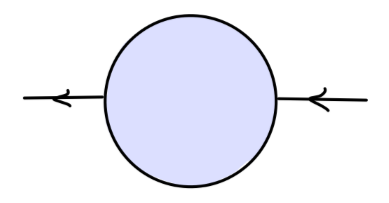$\frac{iZ_2}{\cancel{p}-m}$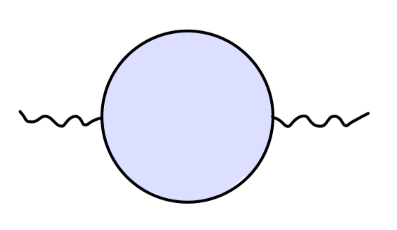$\frac{-iZ_3g_{\mu\nu}}{q^2}$

$\psi = Z_2^{1/2}\psi_r \quad A^{\mu} = Z_3^{1/2} A^{\mu}_r$

$\mathcal{L} = -\frac{1}{4}Z_3(F^{\mu\nu}_r)^2 + Z_2\bar{\psi}_r(i\cancel{\partial}-m_0)\psi_r - Z_2Z_3^{1/2} e_0\bar{\psi}_r\gamma^{\mu}\psi_r A_{r\mu} \tag{12}$

$e_0Z_2Z_3^{1/2} = eZ_1$

$\Gamma^{\mu}(q=0) = \gamma^{\mu}$

$m$ 为物理质量，通过引入如下 counterterms：

\begin{aligned} &\delta_m = Z_2m_0-m\\ &\delta_1 = Z_1 - 1 = \frac{e_0}{e}Z_2Z_3^{1/2}-1\\ &\delta_2 = Z_2-1\\ &\delta_3 = Z_3-1\\ \end{aligned}\tag{13}

\begin{aligned} \mathcal{L} &= -\frac{1}{4}(F^{\mu\nu}_r)^2 + \bar{\psi}_r(i\cancel{\partial}-m)\psi_r - e\bar{\psi}_r\gamma^{\mu}\psi_r A_{r\mu}\\ &\quad -\frac{1}{4}\delta_3(F^{\mu\nu}_r)^2 + \bar{\psi}_r(i\delta_2\cancel{\partial}-\delta_m)\psi_r - e\delta_1\bar{\psi}_r\gamma^{\mu}\psi_r A_{r\mu}\\ \end{aligned}\tag{14}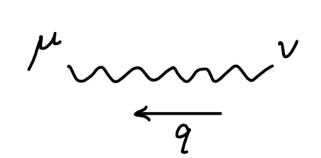$\frac{-ig_{\mu\nu}}{q^2+i\epsilon}$$\frac{i}{\cancel{p}-m+i\epsilon}$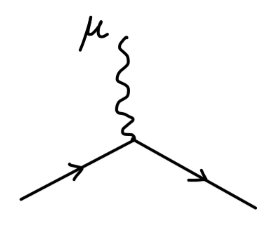$-ie\gamma^{\mu}$$-i(g^{\mu\nu}q^2-q^{\mu}q^{\nu})\delta_3$$i(\cancel{p}\delta_3-\delta_m)$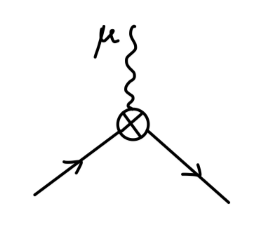$-ie\gamma^{\mu}\delta_1$

1. 光子场强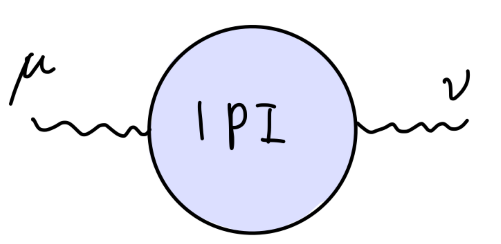$i\Pi^{\mu\nu}(q) = i(g^{\mu\nu}q^2-q^{\mu}q^{\nu})\Pi(q^2)$

1. 电子场强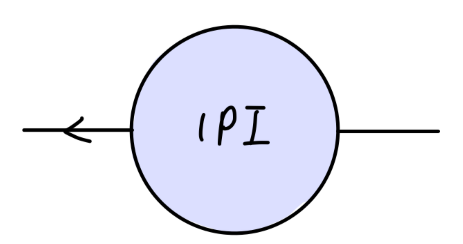$-i\Sigma(\cancel{p})$

1. 电子顶点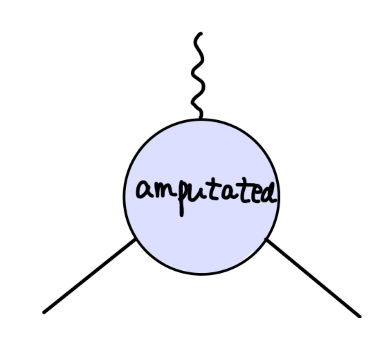$-ie\Gamma^{\mu}(p',p)$

\begin{aligned} \Sigma(\cancel{p}=m)=0\\ \frac{d}{d\cancel{p}}\Sigma(\cancel{p}=m)=0\\ \Pi(q^2=0) =0\\ -ie\Gamma^{\mu}(p'-p=0)= -ie\gamma^{\mu}\\ \end{aligned}\tag{15}

#### QED 重整化理论的一阶圈图计算\begin{aligned} -i\Sigma_2(p) &= -i\frac{e^2}{(4\pi)^{d/2}}\int_{0}^{1}dx\frac{\Gamma(2-\frac{d}{2})}{((1-x)m^2 + x\mu^2 - x(1-x)p^2)^{2-d/2}}\\ &\qquad \times ((4-\epsilon)m - (2-\epsilon)x\cancel{p}) \end{aligned}

$\Sigma(\cancel{p} = m) = \Sigma_2(\cancel{p} = m) - (m\delta_2 - \delta_m) = 0$

\begin{aligned} m\delta_2 - \delta_m &= \Sigma_2(\cancel{p} = m)\\ &= \frac{e^2m}{(4\pi)^{d/2}}\int_{0}^{1}dx\frac{\Gamma(2-\frac{d}{2})\cdot(4-2x-\epsilon(1-x))}{((1-x)^2m^2 + x\mu^2)^{2-d/2}}\\ \end{aligned}\tag{16}

$\frac{d}{d\cancel{p}}\Sigma(\cancel{p}=m)=\frac{d}{d\cancel{p}}\Sigma_2(\cancel{p}=m) - \delta_2 = 0$

\begin{aligned} \delta_2 &= \frac{d}{d\cancel{p}}\Sigma_2(\cancel{p}=m)\\ &= -\frac{e^2}{(4\pi)^{d/2}}\int_{0}^{1}dx\frac{\Gamma(2-\frac{d}{2})}{((1-x)^2m^2 + x\mu^2)^{2-d/2}}\\ &\qquad \times [(2-\epsilon)x - \frac{\epsilon}{2} \frac{2x(1-x)m^2}{(1-x)^2m^2+x\mu^2}(4-2x-\epsilon(1-x))] \end{aligned}\tag{17}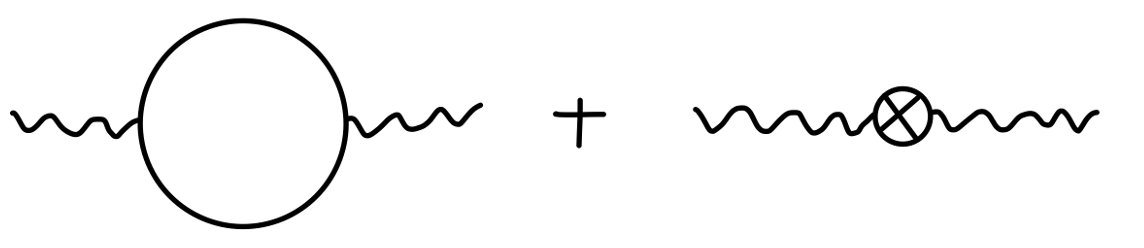$\Pi_2(q^2) = -\frac{e^2}{(4\pi)^{d/2}} \int_{0}^{1}dx \frac{\Gamma(2-\frac{d}{2})}{(m^2-x(1-x)q^2)^{2-d/2}}(8x(1-x))$

$\Pi(q^2=0) = \Pi_2(q^2=0) - \delta_3 = 0$

\begin{aligned} \delta_3 &= \Pi_2(q^2=0)\\ &= -\frac{e^2}{(4\pi)^{d/2}} \int_{0}^{1}dx \frac{\Gamma(2-\frac{d}{2})}{(m^2)^{2-d/2}}(8x(1-x)) \end{aligned}\tag{18}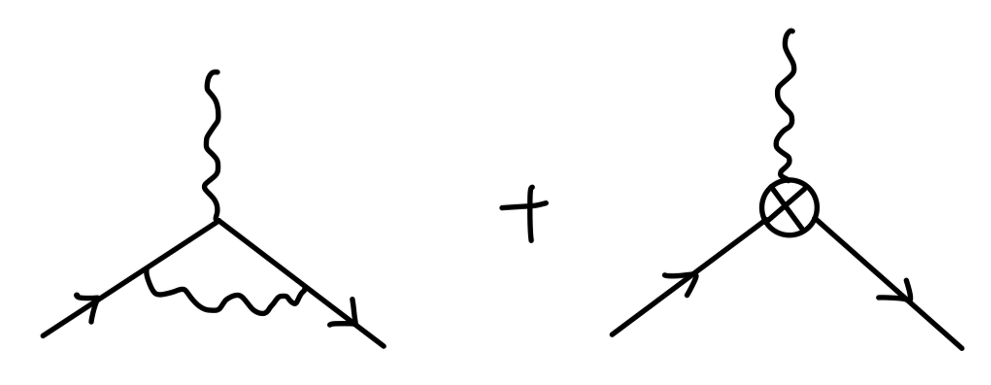\begin{aligned} \delta F_1(q^2) &= \frac{e^2}{(4\pi)^{d/2}} \int dxdydz \delta(x+y+z-1) \{\frac{\Gamma(2-\frac{d}{2})}{\Delta^{2-d/2}}\frac{(2-\epsilon)^2}{2}\\ &\qquad + \frac{\Gamma(3-\frac{d}{2})}{\Delta^{3-d/2}}(q^2[2(1-x)(1-y)-\epsilon xy] + m^2[2(1-4z+z^2) - \epsilon(1-z)^2])\} \end{aligned}

\begin{aligned} -ie\Gamma^{\mu}(p'-p=0) &= -ie\gamma^{\mu}F_1(0) -ie\gamma^{\mu} \delta_1\\ &= -ie\gamma^{\mu}\\ \end{aligned}

\begin{aligned} \delta_1 &= -\delta F_1(0)\\ &= \frac{e^2}{(4\pi)^{d/2}} \int dz(1-z) \{\frac{\Gamma(2-\frac{d}{2})}{((1-z)^2m^2 + z\mu^2)^{2-d/2}}\frac{(2-\epsilon)^2}{2}\\ &\qquad + \frac{\Gamma(3-\frac{d}{2})}{((1-z)^2m^2 + z\mu^2)^{3-d/2}}[2(1-4z+z^2) - \epsilon(1-z)^2]m^2\}\\ \end{aligned}\tag{19}

\begin{aligned} \mathcal{L} &= -\frac{1}{4}(F^{\mu\nu}_r)^2 + \bar{\psi}_r(i\cancel{\partial}-m)\psi_r - e\bar{\psi}_r\gamma^{\mu}\psi_r A_{r\mu}\\ &\quad -\frac{1}{4}\delta_3(F^{\mu\nu}_r)^2 + \bar{\psi}_r(i\delta_2\cancel{\partial}-\delta_m)\psi_r - e\delta_1\bar{\psi}_r\gamma^{\mu}\psi_r A_{r\mu}\\ \end{aligned}\tag{14}

$e = \frac{Z_2}{Z_1}Z_2^{1/2}e_0 = \sqrt{Z_3}e_0$

$Z_1 = Z_2$ 的严格成立是有深刻意义的，比如它保证了对应不同带电轻子，将给出数值相同的电荷量。例如对于 $\mu$ 子：

$e = \frac{Z_2'}{Z_1'}Z_2^{1/2}e_0 = Z_2^{1/2}e_0$